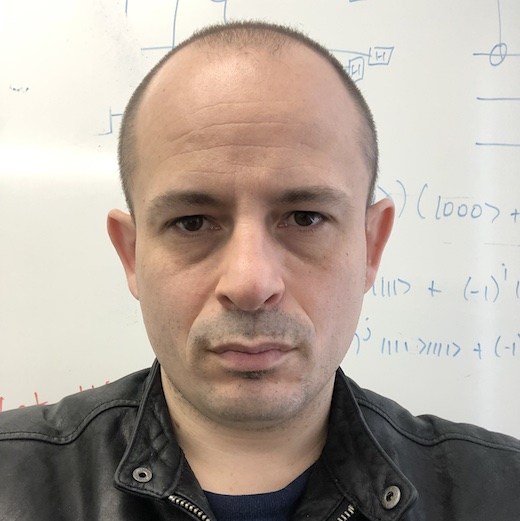## Faculty

Allan Borodin
Complexity Theory,
Online Algorithms,
Game Theory

Faith Ellen
Distributed Complexity,
Data Structures,
Lower Bounds

Distributed Computing

Swastik Kopparty
Complexity Theory,
Error-Correcting Codes, Pseudorandomness

Michael Molloy
Graph Theory,
Combinatorics

Aleksandar Nikolov
Algorithms,
Discrepancy Theory,
Privacy

Toniann Pitassi
Complexity Theory,
Proof Complexity
(On leave)

Benjamin Rossman
Circuit Complexity,
Logic
(On leave)

Sushant Sachdeva
Algorithms,
Optimization,
Learning

Shubhangi Saraf
Complexity Theory,
Algebraic Computation

Nisarg Shah
Mechanism Design,
Game Theory

Sam Toueg
Distributed ComputingNathan Wiebe
Quantum Algorithms,
Quantum Machine Learning

Henry Yuen
Quantum Computing,
Quantum Cryptography,
Complexity Theory
(On leave)

## Emeriti Faculty

Stephen Cook
Complexity Theory,
Logic

Derek Corneil
Graph Theory

Charles Rackoff
Cryptography,
Complexity Theory

Alasdair Urquhart
Complexity of Proofs,
Logic,
History of Logic